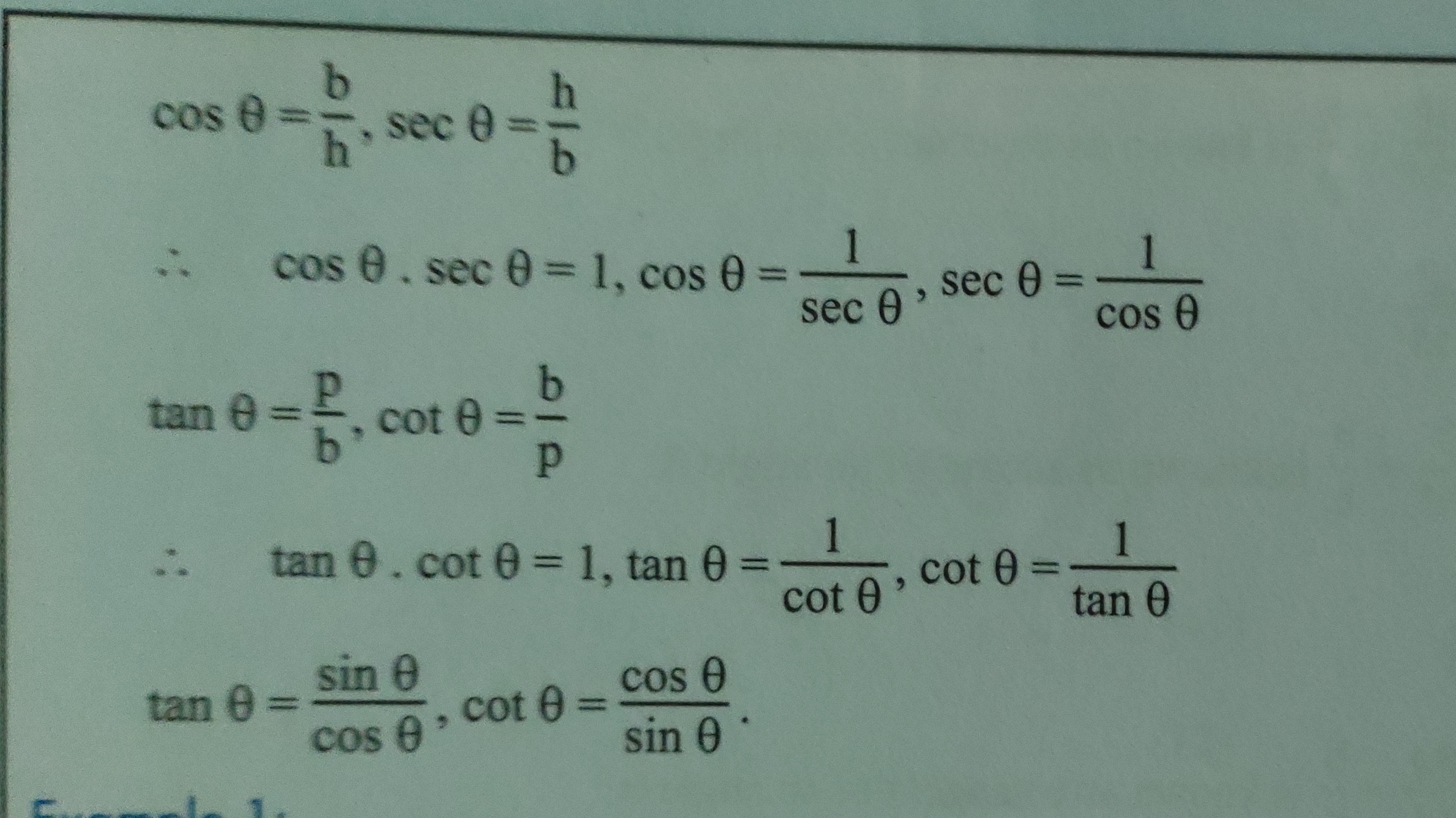Trigonometry:ReviewThe area of mathematics relating to the study of trigonometric function in relation to measurement in triangles is known as trigonometry.

Note:Hypotenuse is the longest side and always in front of the right angle.

Trigonometric Ratios:Relationship Between Trigonometric Ratios:Proofs: